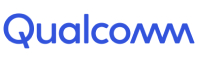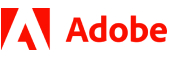New update is available. Click here to update.
Topics

Easy0/40
Average time to solve is 10m+2 more companies

## Problem statement

You are given an integer 'N'. Your task is to find the number formed after swapping each even bit of 'N' in its binary representation with its adjacent bit on the right, assuming that the least significant bit is an odd bit.

For example :

``````Consider the integer N = 45 whose binary representation is 101101. The resulting number formed after swapping each even bit with its adjacent bit to the right will be 30 (011110) in this case.
``````
Detailed explanation ( Input/output format, Notes, Images )
Constraints :
``````1 <= T <= 10^5
1 <= N <= 10^9

Time Limit: 1 sec
``````
Sample Input 1 :
``````2
9
2
``````
Sample Output 1 :
``````6
1
``````
Explanation For Sample Input 1 :
``````For the first test case :
The binary representation of 9 is 1001. Here, we will swap the 2nd bit with the 1st bit and the 4th bit with 3rd bit. The resulting binary number will be 0110. Hence, the answer is 6 in this case.

For the second test case :
The binary representation of 2 is 10. Here, we will swap the 2nd bit with the 1st bit. The resulting binary number will be 01 or 1. Hence, the answer is 1 in this case.
``````
Sample Input 2 :
``````2
7
10
``````
Sample Output 2 :
``````11
5
``````Console